# Bicategory

(diff) ← Older revision | Latest revision (diff) | Newer revision → (diff)

(update), weak-category

A-category [a7], [a8], [a14], [a19], [a22] is a categoryconsisting of objectsand arrowstogether with-arrows, which can be displayed as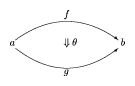Figure: b120230a

and has vertical and horizontal composition operations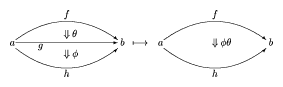Figure: b120230b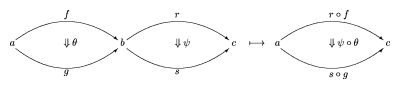Figure: b120230c

These compositions are required to be associative and unital; moreover, horizontal composition must preserve vertical units and the following interchange law is imposed: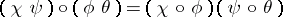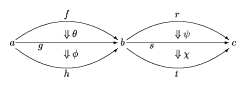Figure: b120230d

The basic example of a-category is, whose objects are (small) categories, whose arrows are the functors (cf. also Functor), and whose-arrows are natural transformations. Indeed, the basic "five rules" for composition of natural transformations appeared in [a11], Appendix.

There is a weaker notion of-category which occurs in practice. A weak-category or bicategory [a1] consists of the data and conditions of a-category except that the associativity and unital equalities for horizontal composition are replaced by the extra data of invertible natural families of-arrows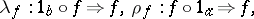called associativity and unital constraints, such that the associativity pentagon (or-cocycle condition)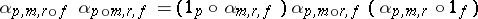and unit triangle (or normalization condition)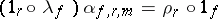are imposed. In some of the recent literature, bicategories are called-categories and-categories are called strict-categories.

A monoidal category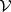can be identified with the one-object bicategory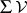whose arrows are the objects of, whose-arrows are the arrows of, whose horizontal composition is the tensor product of, and whose vertical composition is the composition of.

There is a bicategorywhose objects are (small) categories and whose arrows are modules [a31], [a34] (called pro-functors or distributors in [a2], and bimodules in [a21]) between categories.

An arrowin a bicategory is called an equivalence when there is an arrow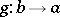such that there are invertible-arrowsand. A weak-groupoid is a bicategory in which each-arrow is invertible and each arrow is an equivalence. A-groupoid is a-category with all arrows and-arrows invertible. For each space, there is a homotopy-groupoid,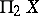whose objects are the points of; it contains the information of the fundamental groupoid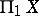and the homotopy groupsfor each(cf. also Homotopy group; Crossed module; Crossed complex; Fundamental group). An early application of-categories to homotopy theory occurs in [a9]. In fact, Ch. Ehresmann [a7] defined double categories and double groupoids, which generalize-categories in that they have two types of arrows (see [a19]), and these also have proved important in homotopy theory [a5].

While many examples occur naturally as bicategories rather than-categories, there is a coherence theorem asserting that every bicategory is equivalent (in the appropriate sense) to a-category [a23], [a12].

There are several purely categorical motivations for the development of bicategory theory. The first is to study bicategories following the theory of categories but taking account of the-dimensionality; this is the spirit of [a10], [a14], [a18], [a29]. A given concept in category theory typically has several generalizations, stemming from the fact that equalities between arrows can be replaced by-arrow constraints (lax generalization), by invertible-arrow constraints (pseudo-generalization), or by keeping the equalities; further equalities are required on the constraints. A bicategory can thus be regarded as a pseudo-category, an equivalence as a pseudo-isomorphism, and a stack (a "champ" in French) as a pseudo-sheaf. In lax cases there are also choices of direction for the equality-breaking constraints. All this applies to functors: there are lax functors (also called morphisms) and pseudo-functors (also called homomorphisms) between bicategories; there are-functors between-categories having equality constraints. This also applies to limits, adjunctions, Kan extensions, etc. [a10], [a14]. One can use the fact that-categories are categories with homs enriched in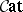, that is,-categories where[a8]. Some laxness is even accounted for in this way: lax limits are enriched limits for a suitable weight (or index) [a28].

A second motivation comes from the fact that bicategories are "monoidal categories with several objects" . Included in this is the study of categories enriched in a bicategory which leads to a unification of category theory, sheaf theory, Boolean-valued logic, and the theory of metric spaces (cf. Metric space) [a38], [a31], [a34], [a3], [a24]. The generalization of Cauchy completion (cf. also Cauchy sequence) from the case of metric spaces is fundamental [a21].

A third impetus is the formalization of properties of the bicategory(as in the part of category theory which abstracts properties of the category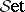of sets) allowing the use of bicategories as organizational tools for studying categories with extra structure (in the way that categories themselves organize sets with structure). This leads to the study of arrow categories [a13], adjunctions [a17], monads (also called triples; cf. Triple) [a26], Kan extensions [a37], [a27] factorization systems (cf. also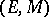-factorization system in a category) [a31], [a32], [a33], [a6], etc., as concepts belonging within a fixed bicategory. Familiar constructions (such as comma categories and Eilenberg–Moore categories for monads) made with these concepts turn out to be limits of the kind arising in other motivations. In this spirit, one can mimic the construction of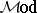fromstarting with a bicategory (satisfying certain exactness conditions) much as one constructs a category of relations in a regular category or topos [a29], [a6], [a25]. The size needs of category theory add extra challenges to the subject [a27], [a37].

Low-dimensional topology enters bicategory theory from two dual directions. The commutative diagrams familiar in a category (cf. also Diagram) laxify in a bicategory to-dimensional diagrams with-arrows in the regions; and these diagrams, if well formed, can be evaluated, using the compositions, to yield a unique-arrow called the pasted composite of the diagram [a1], [a14], [a19]. Two-dimensional graph-like structures called computads were designed to formalize pasting [a28]. The planar Poincaré-dual view replaces pasting diagrams with string diagrams; the-arrows label nodes, the arrows label strings (intervals embedded in the Euclidean plane), and the objects label regions [a16], [a36]. The planar geometry of string diagrams under deformation is faithful to the algebra of bicategories. See also Higher-dimensional category; Bicategory.

How to Cite This Entry:
Bicategory. Ross Street (originator), Encyclopedia of Mathematics. URL: http://encyclopediaofmath.org/index.php?title=Bicategory&oldid=19168
This text originally appeared in Encyclopedia of Mathematics - ISBN 1402006098